# Copper plate

Copper plate length 3.2 m and width 50 cm weighs 55.42 kg. What is the plate thick, if 1 m3 of copper weighs about 8700 kg?

Result

d =  4 mm

#### Solution:Leave us a comment of example and its solution (i.e. if it is still somewhat unclear...):Be the first to comment!#### To solve this example are needed these knowledge from mathematics:

Do you want to convert length units? Do you want to convert mass units?

## Next similar examples:

1. Copper sheetThe copper plate has a length of 1 m, width 94 cm and weighs 9 kg. What is the plate thickness, if 1 m3 weighs 8715 kg?
2. Water lakeThe length of the lake water is 8 meters width 7 meters and depth 120 centimeters. How many liters of water can fit into the water lake?
3. Scale 3Miriam room is 3.2 meters wide. It is draw by line segment length 6.4 cm on floor plan. In what scale it is plan of the room?
4. Trunk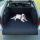Calculate length of the biggest fishing rod can be inserted into the trunk of a car with dimensions 165 x 99 × 85 cm?
5. Feet to milesA student runs 2640 feet. If the student runs an additional 7920 feet, how many total miles does the student run?
6. Customary lengthConvert length 65yd 2 ft to ft
7. Cuboid boxHow many m2 paper is needed for the sticking cuboid box of dimensions 50 cm, 40 cm and 30 cm? To the folds add one-tenth the area.
8. StepsWalkway from the house to the school is 180 m long. How many more steps Michael makes than his father on the way to school, if the length of Michael's step is 60 cm and the length of his father's step is 90 cm.
9. Wood dividingWhich equation calculates the number of 1/3-foot pieces that can be cut from a piece of wood that is 7 feet long?
10. Snowman 3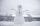During the last winter carnival, the local college students built a 30- foot snowman out of 109 tons of snow. How much snow will be needed to build a 36- foot snowman this year?
11. Liter of goldWhat weight does 1 dm3 of gold have? Gold density is 19,300 kg / m3
12. Stew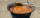To prepare stew for 10 loggers we need: 2.5 kg of potatoes, 0.8 kg of meat, 18 dag of flour, 30 dag onion and 1 bread. What is the required amount of raw materials for 50 participants camps where loggers eats 1.5 times more than a participant of the camp?
13. Wood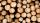Wood density is 0.6 g/cm3. How many kilograms weight 1 m3 of wood?
14. Pound2kilosHow many pounds make 1 kilograms?
15. RecipeA recipe requires 2 pounds of flour. If a chef wants to triple the recipe, how many ounces of flour will be needed?
16. Tank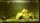The tank bottom has dimensions of 1.5 m and 3 2/6 m. The tank is 459.1 hl of water. How high is the water surface?
17. Excavator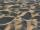The excavator bucket capacity is 0.5 m3. Determine the mass of sand that the excavator picks up. The sand density is 1650 kg/m3.